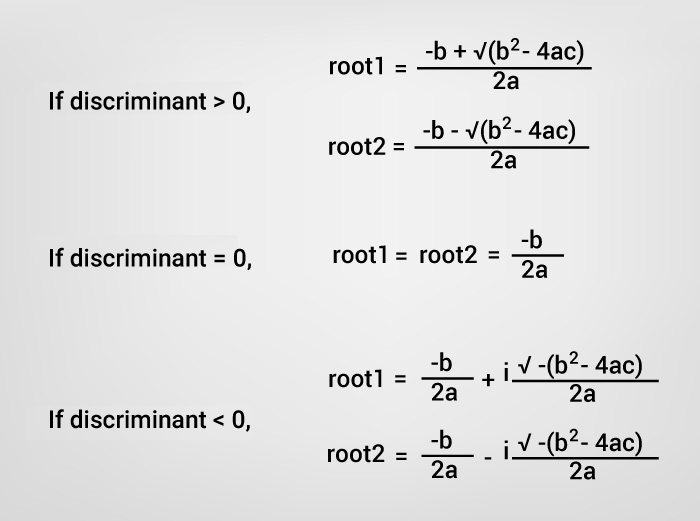# C Program to Find all Roots of a Quadratic Equation

This program accepts coefficients of a quadratic equation from the user and displays the roots (both real and complex roots depending upon the discriminant ).To understand this example, you should have the knowledge of the following C programming topics:

The standard form of a quadratic equation is:

```ax2 + bx + c = 0, where
a, b and c are real numbers and
a ≠ 0
```

The term `b2-4ac` is known as the discriminant of a quadratic equation. The discriminant tells the nature of the roots.

• If discriminant is greater than 0, the roots are real and different.
• If discriminant is equal to 0, the roots are real and equal.
• If discriminant is less than 0, the roots are complex and different.## Example: Program to Find Roots of a Quadratic Equation

``````#include <stdio.h>
#include <math.h>

int main()
{
double a, b, c, discriminant, root1, root2, realPart, imaginaryPart;

printf("Enter coefficients a, b and c: ");
scanf("%lf %lf %lf",&a, &b, &c);

discriminant = b*b-4*a*c;

// condition for real and different roots
if (discriminant > 0)
{
// sqrt() function returns square root
root1 = (-b+sqrt(discriminant))/(2*a);
root2 = (-b-sqrt(discriminant))/(2*a);

printf("root1 = %.2lf and root2 = %.2lf",root1 , root2);
}

//condition for real and equal roots
else if (discriminant == 0)
{
root1 = root2 = -b/(2*a);

printf("root1 = root2 = %.2lf;", root1);
}

// if roots are not real
else
{
realPart = -b/(2*a);
imaginaryPart = sqrt(-discriminant)/(2*a);
printf("root1 = %.2lf+%.2lfi and root2 = %.2f-%.2fi", realPart, imaginaryPart, realPart, imaginaryPart);
}

return 0;
}
``````

Output

```Enter coefficients a, b and c: 2.3
4
5.6
Roots are: -0.87+1.30i and -0.87-1.30i```

In this program, library function `sqrt()` is used to find the square root of a number. To learn more, visit:  sqrt() function.